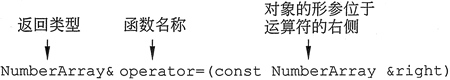## 赋值运算符重载（C++实现）详解

• 内容
• 评论
• 相关

C++ 允许程序员重新定义标准运算符在与类对象一起使用时的工作方式。

NumberArray first(3,10.5);
NumberArray second(5, 20.5);

first = second;

NumberArray second = first; //复制构造函数被调用

second = first; //复制构造函数未被调用

```class NumberArray
{
private:
double *aPtr;
int arraySize;
public:
void operator = (const NumberArray & right) ;//重载运算符
NumberArray(const NumberArray &);
NumberArray(int size, double value);
～NumberArray() { if (arraySize > 0) delete [ ] aPtr; }
void print() const;
void setValue(double value);
};```0条评论